6
Chapter
vMathematics is the art of saying many things in many
different ways. MAXWELLv
6.1 Introduction
In earlier classes, we have studied equations in one variable and two variables and also
solved some statement problems by translating them in the form of equations. Now a
natural question arises: ‘Is it always possible to translate a statement problem in the
form of an equation? For example, the height of all the students in your class is less
than 160 cm. Your classroom can occupy atmost 60 tables or chairs or both. Here we
get certain statements involving a sign ‘<’ (less than), ‘>’ (greater than), ‘’ (less than
or equal) and (greater than or equal) which are known as inequalities.
In this Chapter, we will study linear inequalities in one and two variables. The
study of inequalities is very useful in solving problems in the field of science, mathematics,
statistics, economics, psychology, etc.
6.2 Inequalities
Let us consider the following situations:
(i) Ravi goes to market with ` 200 to buy rice, which is available in packets of 1kg. The
price of one packet of rice is ` 30. If x denotes the number of packets of rice, which he
buys, then the total amount spent by him is ` 30x. Since, he has to buy rice in packets
only, he may not be able to spend the entire amount of ` 200. (Why?) Hence
30x < 200
... (1)
Clearly the statement (i) is not an equation as it does not involve the sign of equality.
(ii) Reshma has ` 120 and wants to buy some registers and pens. The cost of one
register is ` 40 and that of a pen is ` 20. In this case, if x denotes the number of
registers and y, the number of pens which Reshma buys, then the total amount spent by
her is ` (40x + 20y) and we have
40x + 20y 120 ... (2)
LINEAR INEQUALITIES
2020-21LINEAR INEQUALITIES 117
Since in this case the total amount spent may be upto ` 120. Note that the statement (2)
consists of two statements
40x + 20y < 120 ... (3)
and 40x + 20y = 120 ... (4)
Statement (3) is not an equation, i.e., it is an inequality while statement (4) is an equation.
Definition 1
Two real numbers or two algebraic expressions related by the symbol
‘<’, ‘>’, ‘
or ‘ form an inequality
.
Statements such as (1), (2) and (3) above are inequalities.
3 < 5; 7 > 5 are the examples of numerical inequalities while
x < 5; y > 2; x 3, y 4 are some examples of literal inequalities.
3 < 5 < 7 (read as 5 is greater than 3 and less than 7), 3
< x < 5 (read as x is greater
than or equal to 3 and less than 5) and 2 < y < 4 are the examples of double inequalities.
Some more examples of inequalities are:
ax + b < 0 ... (5)
ax + b > 0 ... (6)
ax + b 0 ... (7)
ax + b 0 ... (8)
ax + by < c ... (9)
ax + by
> c ... (10)
ax + by c ... (11)
ax + by c ... (12)
ax
2
+ bx + c 0 ... (13)
ax
2
+ bx + c > 0 ... (14)
Inequalities (5), (6), (9), (10) and (14) are strict inequalities while inequalities (7), (8),
(11), (12), and (13) are slack inequalities. Inequalities from (5) to (8) are linear
inequalities in one variable x when a
0, while inequalities from (9) to (12) are linear
inequalities in two variables x and y when a
0, b
0.
Inequalities (13) and (14) are not linear (in fact, these are quadratic inequalities
in one variable x when a
0).
In this Chapter, we shall confine ourselves to the study of linear inequalities in one
and two variables only.
2020-21118 MATHEMATICS
6.3 Algebraic Solutions of Linear Inequalities in One
Variable and their
Graphical Representation
Let us consider the inequality (1) of Section 6.2, viz, 30x <
200
Note that here x denotes the number of packets of rice.
Obviously, x cannot be a negative integer or a fraction. Left hand side (L.H.S.) of this
inequality is 30x and right hand side (RHS) is 200. Therefore, we have
For x = 0, L.H.S. = 30 (0) = 0 < 200 (R.H.S.), which is true.
For x = 1, L.H.S. = 30 (1) = 30 < 200 (R.H.S.), which is true.
For x = 2, L.H.S. = 30 (2) = 60 < 200, which is true.
For x = 3, L.H.S. = 30 (3) = 90 < 200, which is true.
For x = 4, L.H.S. = 30 (4) = 120 < 200, which is true.
For x = 5, L.H.S. = 30 (5) = 150 < 200, which is true.
For x = 6, L.H.S. = 30 (6) = 180 < 200, which is true.
For x = 7, L.H.S. = 30 (7) = 210 < 200, which is false.
In the above situation, we find that the values of x, which makes the above
inequality a true statement, are 0,1,2,3,4,5,6. These values of x, which make above
inequality a true statement, are called solutions of inequality and the set {0,1,2,3,4,5,6}
is called its solution set.
Thus, any solution of an inequality in one variable is a value of the variable
which makes it a true statement.
We have found the solutions of the above inequality by trial and error method
which is not very efficient. Obviously, this method is time consuming and sometimes
not feasible. We must have some better or systematic techniques for solving inequalities.
Before that we should go through some more properties of numerical inequalities and
follow them as rules while solving the inequalities.
You will recall that while solving linear equations, we followed the following rules:
Rule 1 Equal numbers may be added to (or subtracted from) both sides of an equation.
Rule 2 Both sides of an equation may be multiplied (or divided) by the same non-zero
number.
In the case of solving inequalities, we again follow the same rules except with a
difference that in Rule 2, the sign of inequality is reversed (i.e., ‘<‘ becomes ‘>’,
becomes ‘’ and so on) whenever we multiply (or divide) both sides of an inequality by
a negative number. It is evident from the facts that
3 > 2 while – 3 < – 2,
– 8 < – 7 while (– 8) (– 2) > (– 7) (– 2) , i.e., 16 > 14.
2020-21LINEAR INEQUALITIES 119
Thus, we state the following rules for solving an inequality:
Rule 1 Equal numbers may be added to (or subtracted from) both sides of an inequality
without affecting the sign of inequality.
Rule 2 Both sides of an inequality can be multiplied (or divided) by the same positive
number. But when both sides are multiplied or divided by a negative number, then the
sign of inequality is reversed.
Now, let us consider some examples.
Example 1
Solve 30
x < 200 when
(i) x is a natural number,
(ii)
x is an integer.
Solution
We are given 30 x < 200
or
30 200
30 30
x
<
(Rule 2), i.e., x < 20 / 3.
(i) When x is a natural number, in this case the following values of x make the
statement true.
1, 2, 3, 4, 5, 6.
The solution set of the inequality is {1,2,3,4,5,6}.
(ii) When x is an integer, the solutions of the given inequality are
..., – 3, –2, –1, 0, 1, 2, 3, 4, 5, 6
The solution set of the inequality is {...,–3, –2,–1, 0, 1, 2, 3, 4, 5, 6}
Example 2 Solve 5x – 3 < 3x +1 when
(i) x is an integer, (ii) x is a real number.
Solution We have, 5x –3 < 3x + 1
or 5x –3 + 3 < 3x +1 +3 (Rule 1)
or 5x < 3x +4
or 5x – 3x < 3x + 4 – 3x (Rule 1)
or 2x < 4
or x < 2 (Rule 2)
(i) When x is an integer, the solutions of the given inequality are
..., – 4, – 3, – 2, – 1, 0, 1
(ii) When x is a real number, the solutions of the inequality are given by x < 2,
i.e., all real numbers x which are less than 2. Therefore, the solution set of
the inequality is x (– , 2).
We have considered solutions of inequalities in the set of natural numbers, set of
integers and in the set of real numbers. Henceforth, unless stated otherwise, we shall
solve the inequalities in this Chapter in the set of real numbers.
2020-21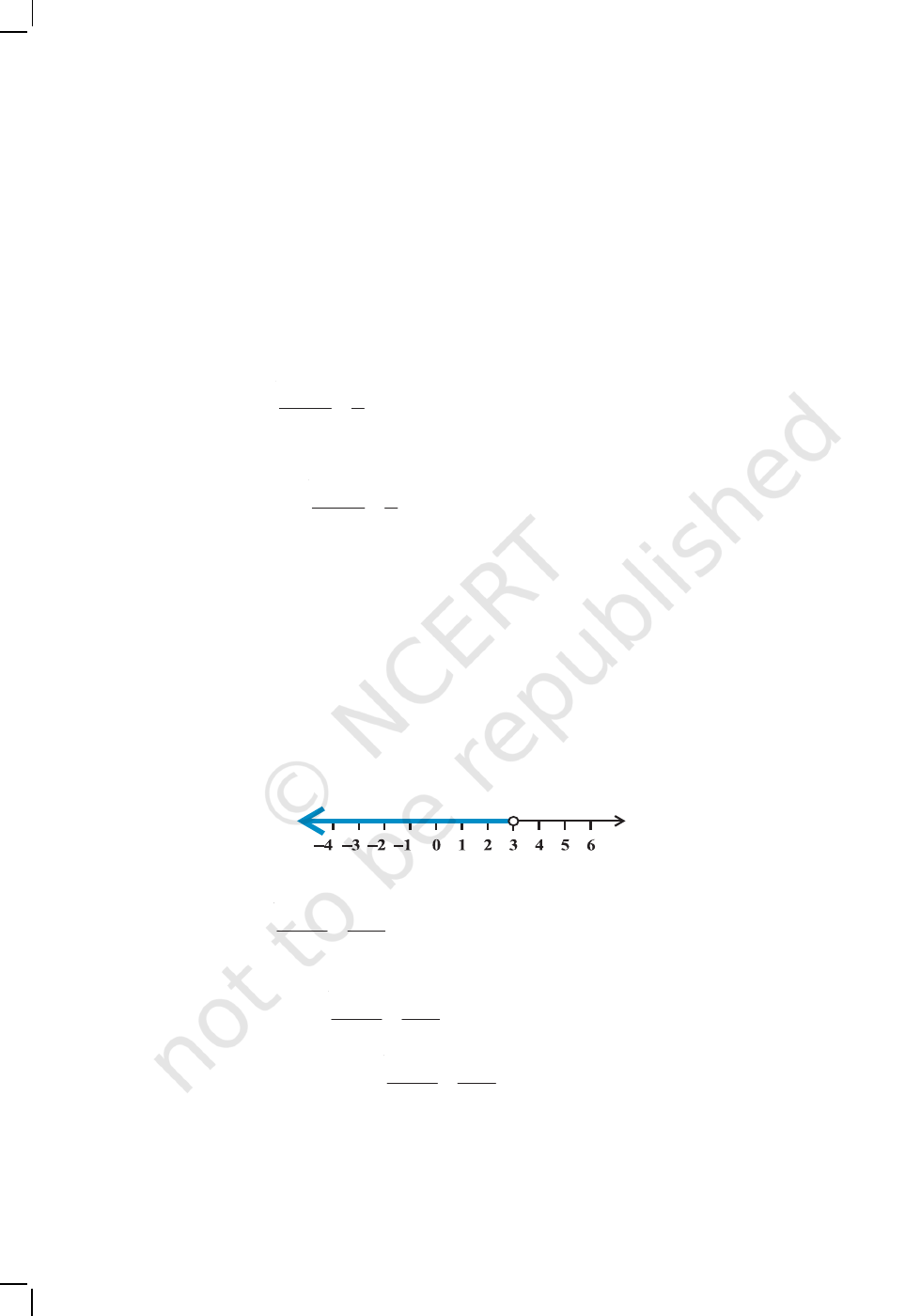120 MATHEMATICS
Example 3 Solve 4x + 3 < 6x +7.
Solution
We have, 4x + 3 < 6x + 7
or 4x – 6x < 6x + 4 – 6
x
or – 2x < 4 or
x > – 2
i.e., all the real numbers which are greater than –2, are the solutions of the given
inequality. Hence, the solution set is (–2, ∞).
Example 4 Solve
5 2
5
3 6
x x
.
Solution We have
5 2
5
x x
or 2 (5 – 2x) x – 30.
or 10 – 4x x – 30
or – 5x – 40, i.e., x 8
Thus, all real numbers x which are greater than or equal to 8 are the solutions of the
given inequality, i.e., x [8, ).
Example 5 Solve 7x + 3 < 5x + 9. Show the graph of the solutions on number line.
Solution We have 7x + 3 < 5x + 9 or
2
x < 6 or x < 3
The graphical representation of the solutions are given in Fig 6.1.
Fig 6.1
Example 6 Solve
3 4 1
1
2 4
x x
+
. Show the graph of the solutions on number line.
Solution We have
3 4 1
1
2 4
x x +
or
3 4 3
x x
or 2 (3x – 4) (x – 3)
2020-21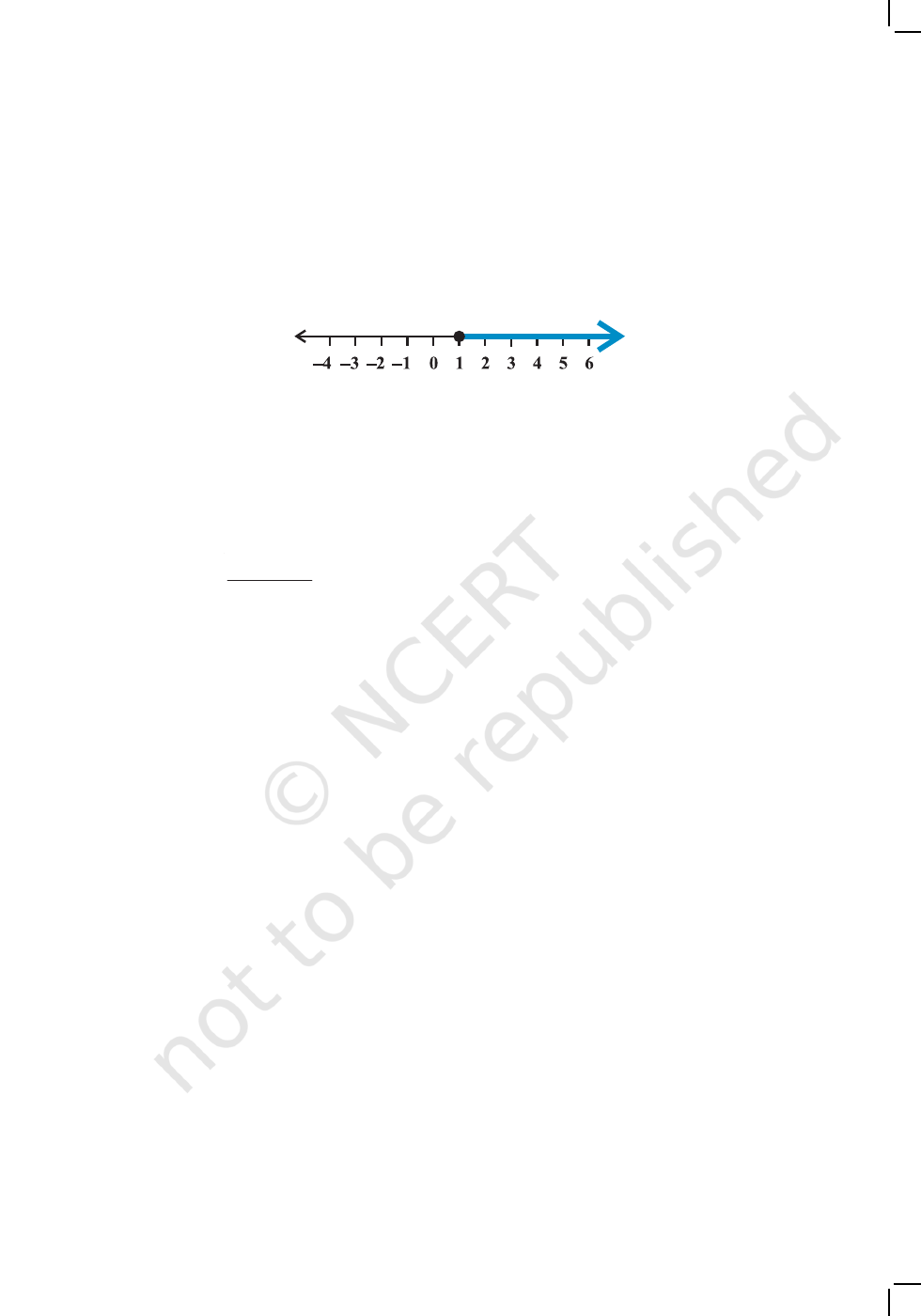LINEAR INEQUALITIES 121
or 6x – 8 x – 3
or 5x 5 or x 1
The graphical representation of solutions is given in Fig 6.2.
Fig 6.2
Example 7 The marks obtained by a student of Class XI in first and second terminal
examination are 62 and 48, respectively. Find the minimum marks he should get in the
annual examination to have an average of at least 60 marks.
Solution Let x be the marks obtained by student in the annual examination. Then
62 48
60
3
x
+ +
or 110 + x 180
or x 70
Thus, the student must obtain a minimum of 70 marks to get an average of at least
60 marks.
Example 8 Find all pairs of consecutive odd natural numbers, both of which are larger
than 10, such that their sum is less than 40.
Solution Let x be the smaller of the two consecutive odd natural number, so that the
other one is x +2. Then, we should have
x > 10 ... (1)
and x + ( x + 2) < 40 ... (2)
Solving (2), we get
2x + 2 < 40
i.e., x < 19 ... (3)
From (1) and (3), we get
10 < x < 19
Since x is an odd number, x can take the values 11, 13, 15, and 17. So, the required
possible pairs will be
(11, 13), (13, 15), (15, 17), (17, 19)
2020-21122 MATHEMATICS
EXERCISE 6.1
1. Solve 24
x < 100, when
(i) x is a natural number. (ii) x is an integer.
2. Solve – 12
x > 30, when
(i) x is a natural number. (ii) x is an integer.
3. Solve 5x – 3 < 7, when
(i) x is an integer. (ii) x is a real number.
4. Solve 3
x + 8 >2, when
(i) x is an integer. (ii) x is a real number.
Solve the inequalities in Exercises 5 to 16 for real x.
5. 4x + 3 < 5
x + 7 6. 3x – 7 > 5x – 1
7. 3(x – 1) 2 (x – 3) 8. 3 (2 –
x) 2 (1 – x)
9.
11
2 3
x x
x
+ + <
10.
1
3 2
x x
> +
11.
3( 2) 5(2 )
5 3
x x
12.
1 3 1
4 ( 6)
2 5 3
x
x
+
13. 2 (2x + 3) – 10 < 6 (x – 2) 14. 37 – (3x + 5) > 9x – 8 (x – 3)
15.
(5 2) (7 3)
4 3 5
x x x
<
16.
(2 1) (3 2) (2 )
3 4 5
x x x
Solve the inequalities in Exercises 17 to 20 and show the graph of the solution in each
case on number line
17. 3x – 2 < 2x + 1 18. 5x – 3 > 3x – 5
19. 3 (1 – x) < 2 (x + 4) 20.
(5 2) (7 3)
2 3 5
x x x
21. Ravi obtained 70 and 75 marks in first two unit test. Find the minimum marks he
should get in the third test to have an average of at least 60 marks.
22. To receive Grade ‘A in a course, one must obtain an average of 90 marks or
more in five examinations (each of 100 marks). If Sunita’s marks in first four
examinations are 87, 92, 94 and 95, find minimum marks that Sunita must obtain
in fifth examination to get grade ‘A in the course.
23. Find all pairs of consecutive odd positive integers both of which are smaller than
10 such that their sum is more than 11.
24. Find all pairs of consecutive even positive integers, both of which are larger than
5 such that their sum is less than 23.
2020-21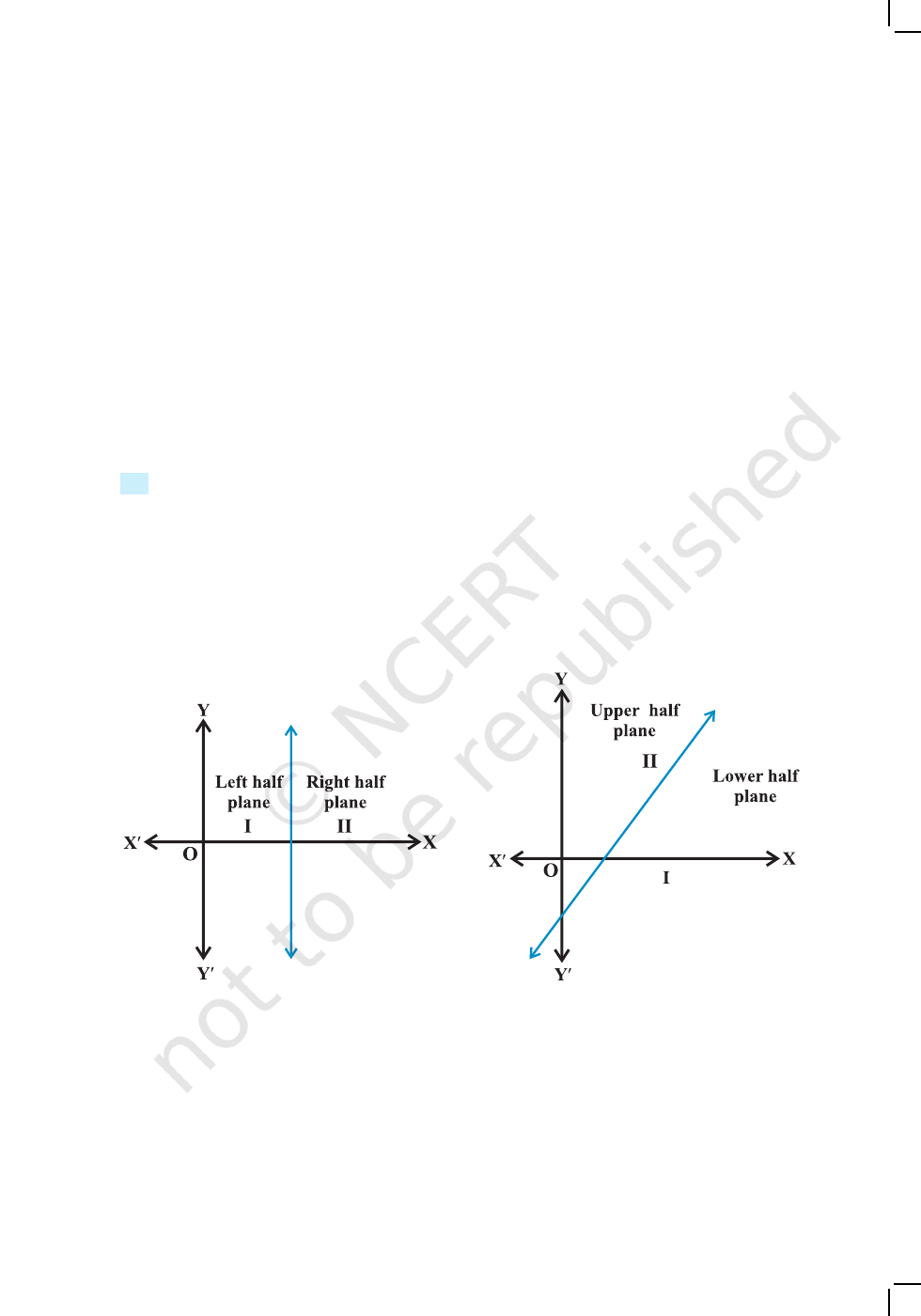LINEAR INEQUALITIES 123
Fig 6.3 Fig 6.4
25. The longest side of a triangle is 3 times the shortest side and the third side is 2 cm
shorter than the longest side. If the perimeter of the triangle is at least 61 cm, find
the minimum length of the shortest side.
26. A man wants to cut three lengths from a single piece of board of length 91cm.
The second length is to be 3cm longer than the shortest and the third length is to
be twice as long as the shortest. What are the possible lengths of the shortest
board if the third piece is to be at least 5cm longer than the second?
[Hint: If
x is the length of the shortest board, then x , (x + 3) and 2x are the
lengths of the second and third piece, respectively. Thus, x + (x + 3) + 2
x 91 and
2x (x + 3) + 5].
6.4 Graphical Solution of Linear Inequalities in Two Variables
In earlier section, we have seen that a graph of an inequality in one variable is a visual
representation and is a convenient way to represent the solutions of the inequality.
Now, we will discuss graph of a linear inequality in two variables.
We know that a line divides the Cartesian plane into two parts. Each part is
known as a half plane. A vertical line will divide the plane in left and right half planes
and a non-vertical line will divide the plane into lower and upper half planes
(Figs. 6.3 and 6.4).
A point in the Cartesian plane will either lie on a line or will lie in either of the half
planes I or II. We shall now examine the relationship, if any, of the points in the plane
and the inequalities ax + by <
c or ax + by > c.
Let us consider the line
ax + by = c, a 0, b 0 ... (1)
2020-21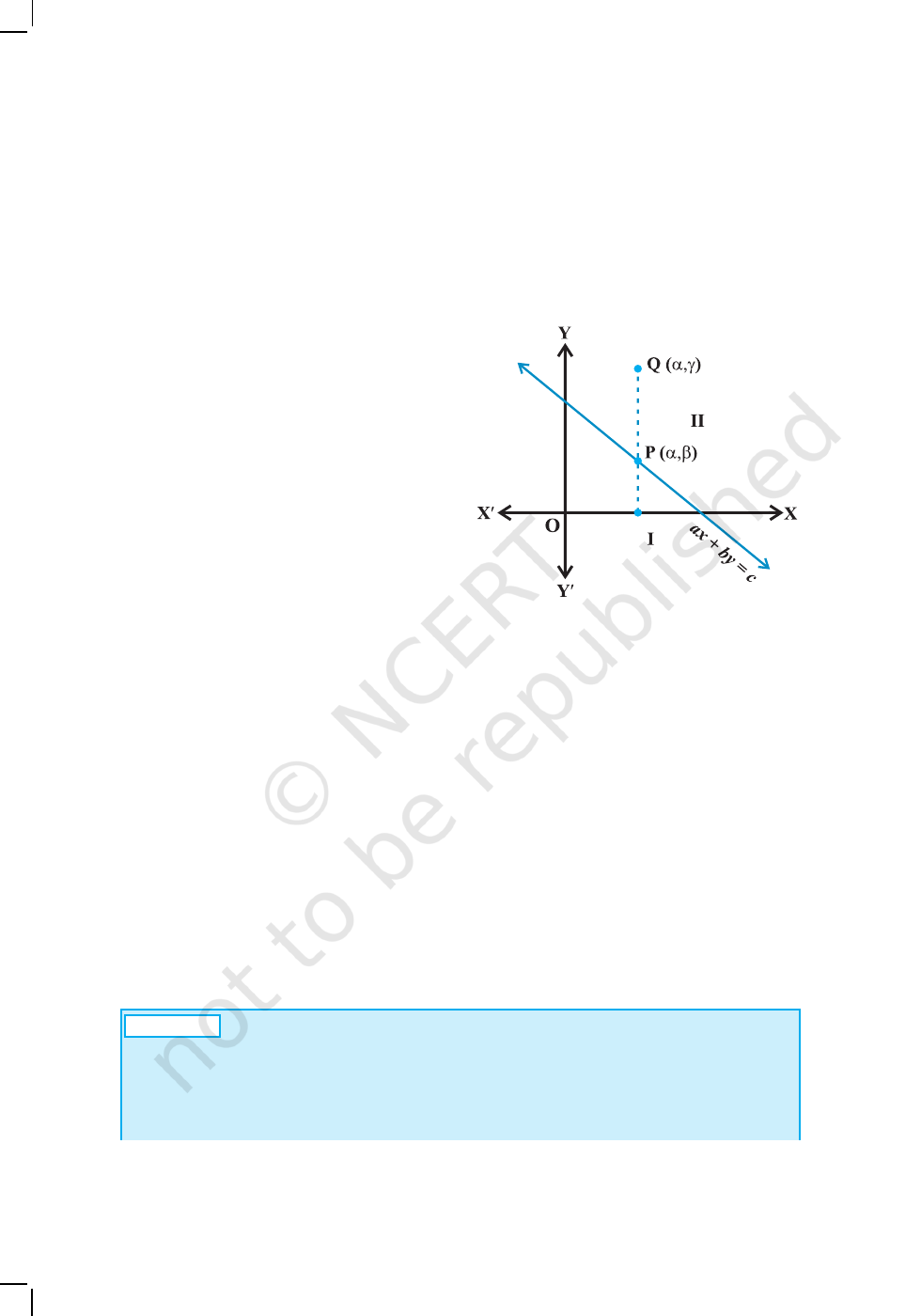124 MATHEMATICS
Fig 6.5
There are three possibilities namely:
(i) ax + by = c (ii) ax + by > c (iii) ax + by < c.
In case (i), clearly, all points (x, y) satisfying (i) lie on the line it represents and
conversely. Consider case (ii), let us first assume that b > 0. Consider a point P (α,β)
on the line ax + by = c, b > 0, so that
aα
+ bβ = c.
Take an arbitrary point
Q (α , γ) in the half plane II (Fig 6.5).
Now, from Fig 6.5, we interpret,
γ > β (Why?)
or b
γ
> bβ or aα + b γ > aα + bβ
(Why?)
or aα + b γ > c
i.e., Q(α,
γ
) satisfies the inequality
ax + by > c.
Thus, all the points lying in the half
plane II above the line ax + by = c satisfies
the inequality ax + by > c. Conversely, let (α, β) be a point on line ax + by = c and an
arbitrary point Q(α, γ) satisfying
ax + by > c
so that aα + bγ > c
aα + b γ > aα + bβ (Why?)
γ > β (as b > 0)
This means that the point (α,
γ
) lies in the half plane II.
Thus, any point in the half plane II satisfies ax + by > c, and conversely any point
satisfying the inequality ax + by > c lies in half plane II.
In case b < 0, we can similarly prove that any point satisfying ax + by > c lies in
the half plane I, and conversely.
Hence, we deduce that all points satisfying ax + by > c lies in one of the half
planes II or I according as b > 0 or b < 0, and conversely.
Thus, graph of the inequality ax + by > c will be one of the half plane (called
solution region) and represented by shading in the corresponding half plane.
Note 1 The region containing all the solutions of an inequality is called the
solution region.
2. In order to identify the half plane represented by an inequality, it is just sufficient
to take any point (a, b) (not online) and check whether it satisfies the inequality or
not. If it satisfies, then the inequality represents the half plane and shade the region
A
2020-21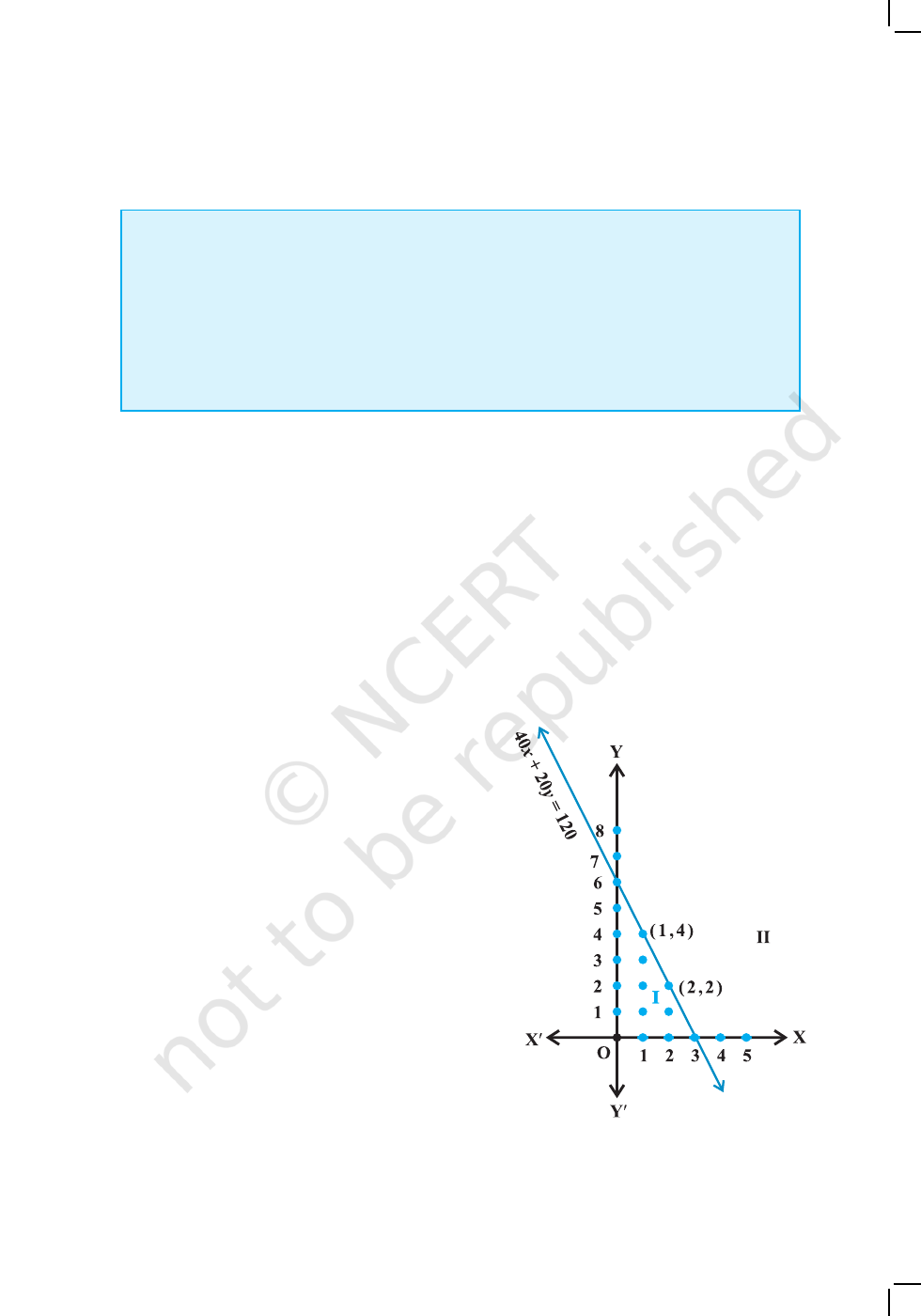LINEAR INEQUALITIES 125
Fig 6.6
which contains the point, otherwise, the inequality represents that half plane which
does not contain the point within it. For convenience, the point (0, 0) is preferred.
3. If an inequality is of the type ax + by c or ax + by c, then the points on the
line ax + by = c are also included in the solution region. So draw a dark line in the
solution region.
4. If an inequality is of the form ax + by
> c or
ax + by < c, then the points on the
line ax + by = c are not to be included in the solution region. So draw a broken or
dotted line in the solution region.
In Section 6.2, we obtained the following linear inequalities in two variables
x and y: 40x + 20y 120 ... (1)
while translating the word problem of purchasing of registers and pens by Reshma.
Let us now solve this inequality keeping in mind that x and y can be only whole
numbers,
since the number of articles cannot be a fraction or a negative number. In
this case, we find the pairs of values of x and y, which make the statement (1) true. In
fact, the set of such pairs will be the solution set of the inequality (1).
x = 0. Then L.H.S. of (1) is
40x + 20y = 40 (0) + 20
y = 20y.
Thus, we have
20y 120 or y 6 ... (2)
For x = 0, the corresponding values of y can be 0, 1, 2, 3, 4, 5, 6 only. In this case, the
solutions of (1) are (0, 0), (0, 1), (0,2), (0,3), (0,4),
(0, 5) and (0, 6).
Similarly, other solutions of (1), when
x = 1, 2 and 3 are: (1, 0), (1, 1), (1, 2), (1,
3), (1, 4), (2, 0), (2, 1), (2, 2), (3, 0)
This is shown in Fig 6.6.
Let us now extend the domain of x and y
from whole numbers to real numbers, and see
what will be the solutions of (1) in this case.
You will see that the graphical method of solution
will be very convenient in this case. For this
purpose, let us consider the (corresponding)
equation and draw its graph.
40x + 20y = 120 ... (3)
In order to draw the graph of the inequality
(1), we take one point say (0, 0), in half plane I
and check whether values of x and y satisfy the
inequality or not.
2020-21